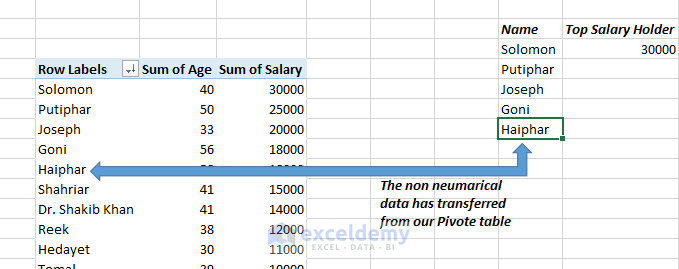Disclosure: This post may contain affiliate links, meaning when you click the links and make a purchase, we receive a commission.

# How to Use Pivot Table Data in Excel Formulas

In this article, I will show you how you can use Pivot Table data in Excel formulas.

No data analyst can think a day without using Pivot Table in their day to day data analysis job. Pivot Table is one of the sophisticated parts of Microsoft Excel. Using pivot table, you can slice and dice your data within few minutes. Without pivot table, same data analysis jobs might take several hours or days.

## How GETPIVOTDATA() works with cell reference

After creating a pivot table, if we select a cell within the pivot table to use it in an Excel formula, GETPIVOTDATA function is automatically inserted in the formula. Let’s see it with an example.

Note: you can also put off the GETPIVOTDATA function to be automatically used.

At first, we need to create a pivot table. In the picture below, you are seeing a pivot table that I have created already: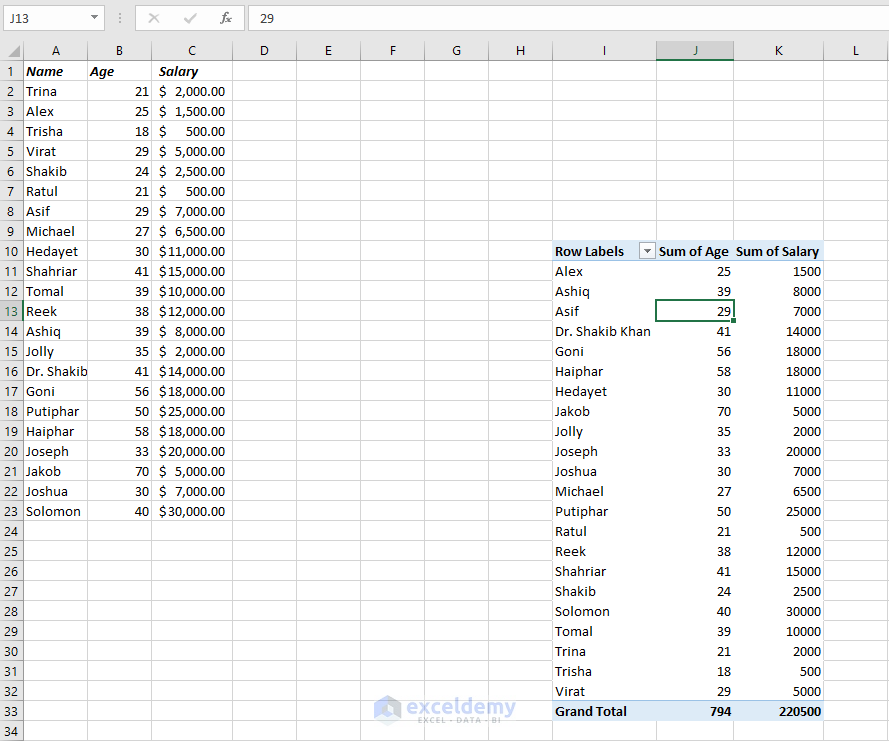Now I will show you how to reference a pivot table cell within an Excel formula.

Somewhere in the same worksheet, I put the “Name” and the “Top Salary Holder” headings in the Excel sheet.

Related: Data Analysis with Excel Pivot Tables (Affiliate Link)

Under the Name, I input manually “Solomon”, a name from the pivot table. I want to show his salary in the right-side cell.

Let’s see how we can do that.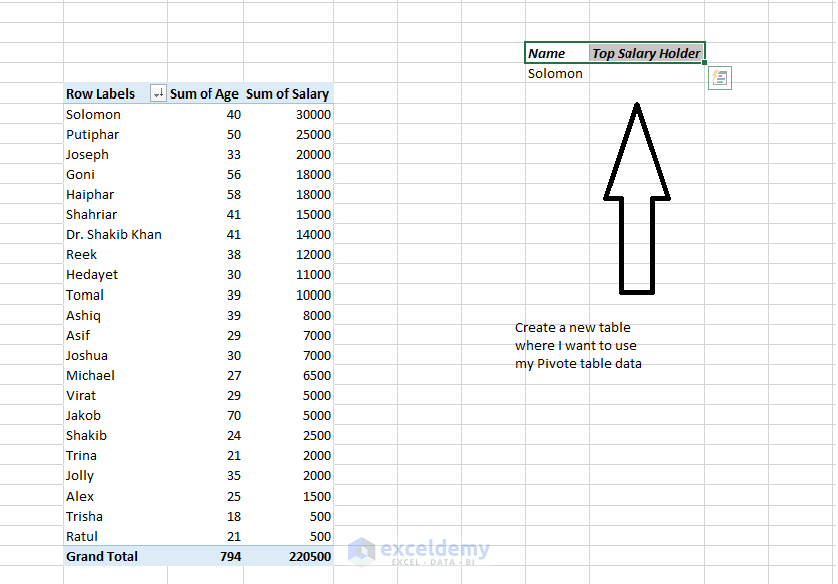Now, I input ‘=’ as shown in the picture below: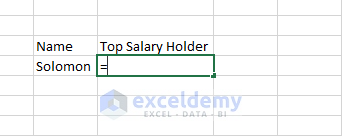Then I select the cell in the pivot table. You see, GETPIVOTDATA() function is automatically inserted into the formula.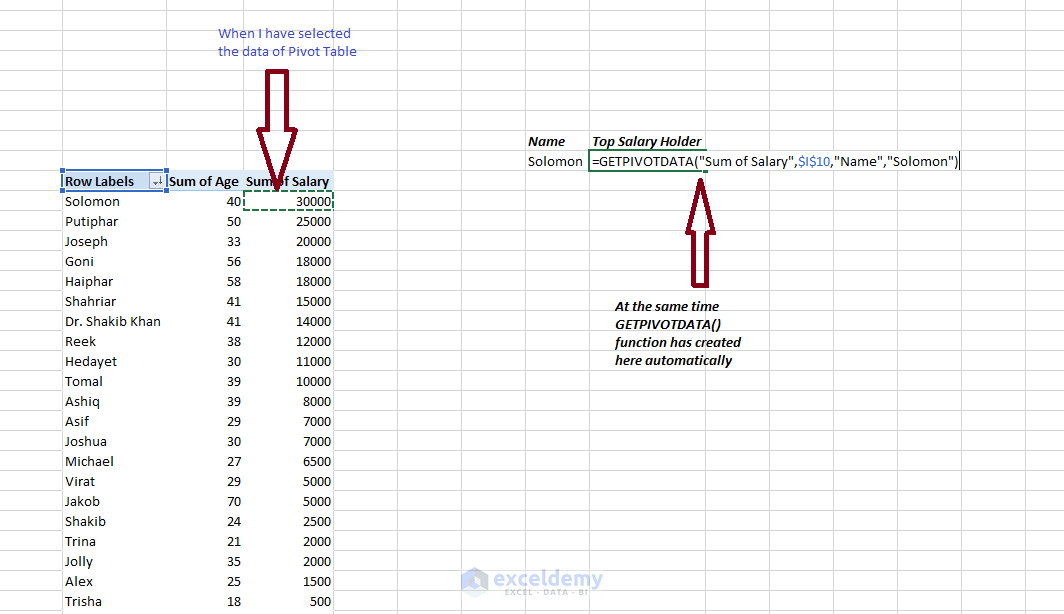Then I just press Enter key on the keyboard. In the picture below, you see the data “30,000” is showing in the cell.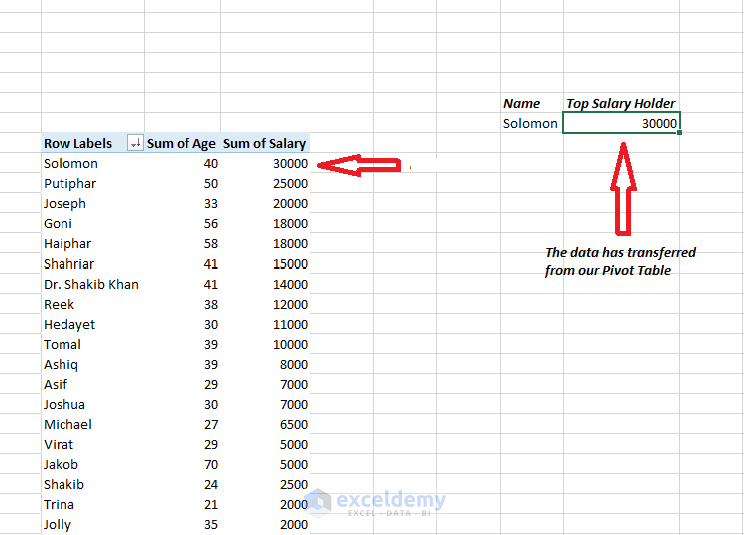This is how you can use GETPIVOTDATA() function to reference a cell in the pivot table.

## How to Use GETPIVOTDATA() Function Dynamically

In our last example, you have seen we got a formula with GETPIVOTDATA function like this:

=GETPIVOTDATA(“Sum of Salary”,\$I\$10,”Name”,”Solomon“)

This formula is fixed. If you copy the formula down (using Auto Fill), it will only show the value of Solomon’s Sum of Salary.

Now, how you can make this formula dynamic?

If we want to use the function GETPIVOTDATA() dynamically, we need to write this formula in a bit different way.

For example, we can replace “Solomon” with the cell reference “O14”.

Here is our formula that only referred to “Solomon”:

=GETPIVOTDATA(“Sum of Salary”,\$I\$10,”Name”,”Solomon“)

And this is our formula now that will work as a dynamic formula:

=GETPIVOTDATA(“Sum of Salary”,\$I\$10,”Name”, O14)

Then we can replace dynamically which can make our work easier.

In the picture below, we will see the replacement process.In the above picture, I want to replace “Solomon” by another name and also replace the salary. To do that we do not need to remove and write again the new name and his salary. We just select the cell “Solomon” and input an equal sign (“=”) and select the Name from pivot table which I want to import by replacing the name “Solomon”.

Read More: Excel Pivot Table Calculated Field (How to Insert & Edit)

Let’s see the below picture: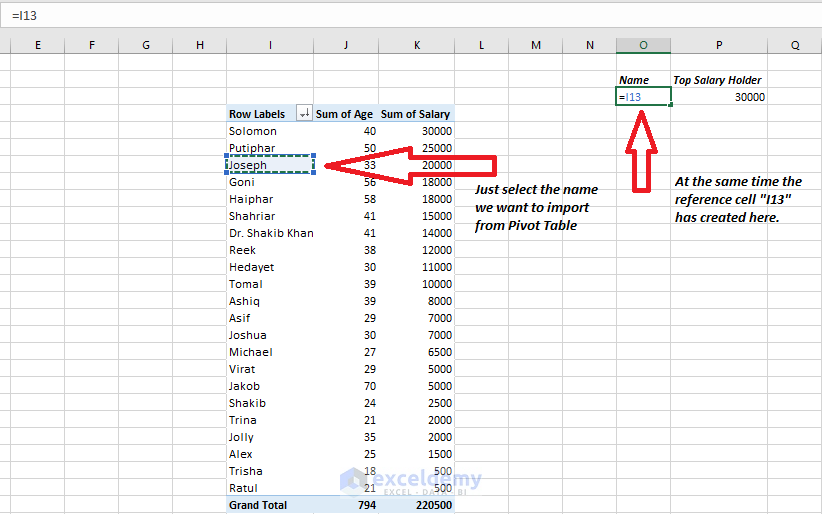Then press the Enter key and we can see the new Name has been imported from the pivot table and also the salary has automatically replaced.

Let’s see the picture below.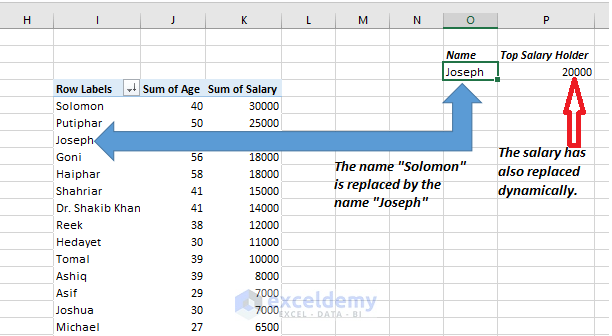Important note:

There is a restriction that we cannot use the GETPIVOTDATA() function for referring non-numeric data and date in a pivot table.

If you want to refer the date and non-numeric data from the pivot table, there is a simple way. Below I’m showing how to refer a non-numeric data from Pivot table.

At first, we input equal sign (‘=’) in a cell like the picture below:Then we select a pivot table cell (I15) that has non-numeric value. See the picture below.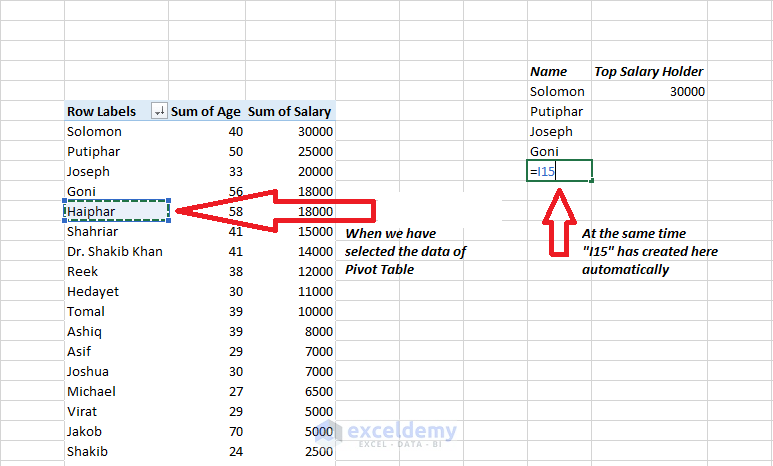Now just press Enter and the data of cell I15 is showing now in cell. See the picture below: